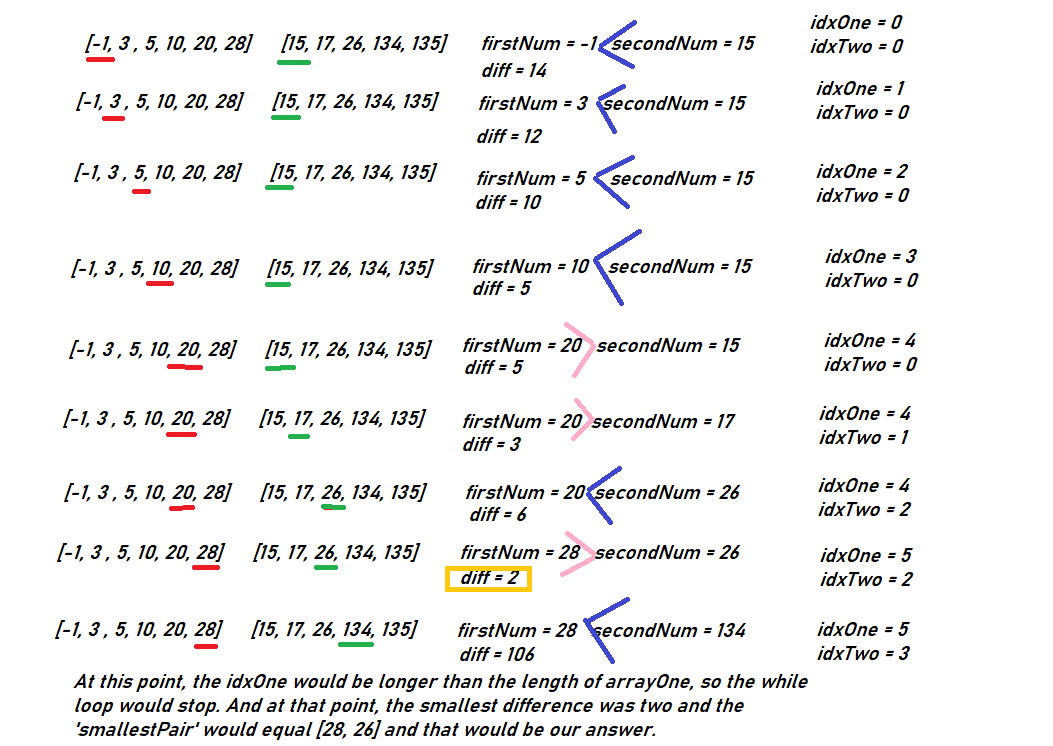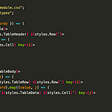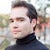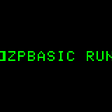# CoDEVIANT #17 (10/3/20) — Pick T̶h̶r̶e̶e̶ Two, M’Lord

`[valueFromArray1, valueFromArray2]`
`function smallestDifference(arrayOne, arrayTwo) { let unoCounter = 0 let dosCounter = 0 let computedValue = null   for(let i = 0; i < arrayOne.length; i ++) {  arrayTwo.forEach((el, index) => {   let value = Math.abs(el - arrayOne[i])   if(computedValue === null || value < computedValue) {    computedValue = value    unoCounter = i    dosCounter = index   }  }) } return [ arrayOne[unoCounter], arrayTwo[dosCounter] ]}`
`let unoCounter = 0let dosCounter = 0let computedValue = null`
`for(let i = 0; i < arrayOne.length; i++) { .....}`
`for(let i = 0; i < arrayOne.length; i++) {   arrayTwo.forEach(el => {      ...   })}`
`for(let i = 0; i < arrayOne.length; i++) {   arrayTwo.forEach(el => {      let value = Math.abs(el - arrayOne[i])   })}`
• set computedValue to equal whatever value is
• set unoCounter to equal whatever i from the classic for-loop is
• set dosCounter to equal index from the use of the forEach-array method ((When you use forEach the first argument, el, in our case, is the value of the array-element. The second argument, index, in our case, is the array-index where the value is located))
`for(let i = 0; i < arrayOne.length; i++) {   arrayTwo.forEach(el => {      let value = Math.abs(el - arrayOne[i])      if(computedValue === null || value < computedValue) {         computedValue = value         unoCounter = i         dosCounter = index      }   })}`
`return [ arrayOne[unoCounter], arrayTwo[dosCounter] ]`
`function smallestDifference(arrayOne, arrayTwo) { let unoCounter = 0 let dosCounter = 0 let computedValue = null   for(let i = 0; i < arrayOne.length; i ++) {  arrayTwo.forEach((el, index) => {   let value = Math.abs(el - arrayOne[i])   if(computedValue === null || value < computedValue) {    computedValue = value    unoCounter = i    dosCounter = index   }  }) } return [ arrayOne[unoCounter], arrayTwo[dosCounter] ]}`
`function smallestDifference(arrayOne, arrayTwo) { arrayOne.sort((a,b) => a-b) arrayTwo.sort((a,b) => a-b) let idxOne = 0 let idxTwo = 0 let smallest = Infinity let current = Infinity let smallestPair = [] while(idxOne < arrayOne.length && idxTwo < arrayTwo.length){  let firstNum = arrayOne[idxOne]  let secondNum = arrayTwo[idxTwo]    if(firstNum < secondNum){    current = secondNum - firstNum    idxOne++  } else if(secondNum < firstNum){    current = firstNum - secondNum    idxTwo++  } else {    return [firstNum, secondNum]  }  if(smallest > current) {    smallest = current    smallestPair = [firstNum, secondNum]  } }  return smallestPair}`
`arrayOne.sort((a,b)=> a - b)arrayTwo.sort((a,b)=> a - b)`
• idxOne: the counter for arrayOne; set to 0
• idxTwo: the counter for arrayTwo; set to 0
• smallest: to keep track of the smallest difference; initially set to Infinity so that no matter what the first difference between values is, it is smaller.
• current: to keep track of the current difference between the two values presently at play; initially set to Infinity as well
• smallestPair: an empty array that will hold the appropriate value from each of the arrays; this is the answer we will return in our function
`let idxOne = 0let idxTwo = 0let smallest = Infinitylet current = Infinitylet smallestPair = []`
`while(idxOne < arrayOne.length && idxTwo < arrayTwo.length) {   ...}`
• firstNum: the value at arrayOne at the position indicated by idxOne
• secondNum: the value at arrayTwo at the position indicated by idxTwo
`while(idxOne < arrayOne.length && idxTwo < arrayTwo.length) {   let firstNum = arrayOne[idxOne]   let secondNum = arrayTwo[idxTwo]}`
`while(idxOne < arrayOne.length && idxTwo < arrayTwo.length) {   let firstNum = arrayOne[idxOne]   let secondNum = arrayTwo[idxTwo]   if(firstNum < secondNum) {     current = secondNum - firstNum     idxOne++   } else if(secondNum < firstNum){     current = firstNum - secondNum     idxTwo++   } else {     return [firstNum, secondNum]   }   if(smallest > current) {     smallest = current     smallestPair = [firstNum, secondNum]   }}`
• arrayOne = [-1, 3, 5, 10, 20, 28]
• arrayTwo = [15, 17, 26, 134, 135]This is basically how our algorithm works with the while loop requiring that idxOne and idxTwo never get larger than the lengths of their arrays.
`function smallestDifference(arrayOne, arrayTwo) { arrayOne.sort((a,b) => a-b) arrayTwo.sort((a,b) => a-b)let idxOne = 0 let idxTwo = 0 let smallest = Infinity let current = Infinity let smallestPair = []while(idxOne < arrayOne.length && idxTwo < arrayTwo.length){  let firstNum = arrayOne[idxOne]  let secondNum = arrayTwo[idxTwo]    if(firstNum < secondNum){    current = secondNum - firstNum    idxOne++  } else if(secondNum < firstNum){    current = firstNum - secondNum    idxTwo++  } else {    return [firstNum, secondNum]  }if(smallest > current) {    smallest = current    smallestPair = [firstNum, secondNum]  } }  return smallestPair}`

--

--

--

is a web developer, opera singer, actor, and lover of cats. (adrian-rosales.tech)

Love podcasts or audiobooks? Learn on the go with our new app.

## Modern JavaScript! 👩🏻‍💻## Use case of Singleton with Axios and TypeScript## Serverless Progressive Web Apps using React with Cloudflare Workers## How imports work in React## Sequelize Database Connection with Nodejsis a web developer, opera singer, actor, and lover of cats. (adrian-rosales.tech)

## BASIC Necessities## Introduction to Git## Fetching Script Tags and Attributes from String## What is S.O.L.I.D Principle?